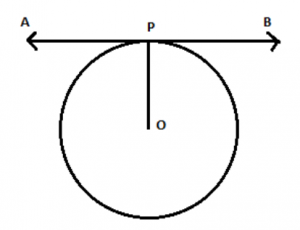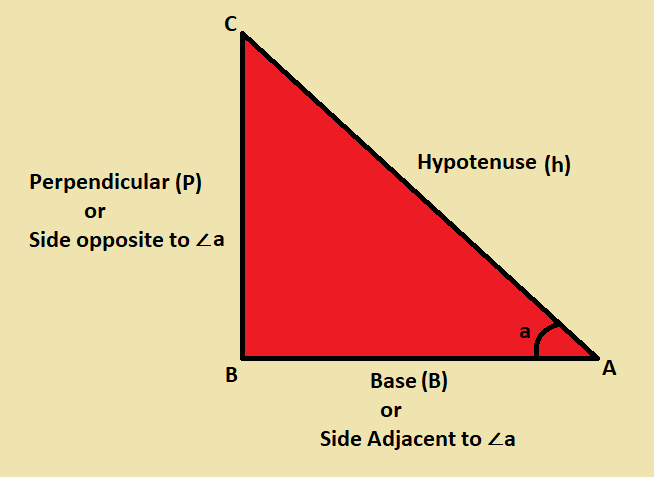# Tangent

## Tangent Meaning in Geometry

In Geometry, the tangent is defined as a line touching circles or an ellipse at only one point. Suppose a line touches the curve at P, then the point “P” is called the point of tangency. In other words, it is defined as the line which represents the slope of a curve at that point. The tangent equation in differential geometry can be found using the following procedures:

As we know that the gradient of the curve is equal to the gradient of the tangent to the curve at any point given on the curve. We can find the tangent equation of the curve y = f(x) as follows:

• Find the derivative of gradient function using the differentiation rules
• To calculate the gradient of the tangent, substitute the x- coordinate of the given point in the derivative
• In the straight-line equation (in a slope-point formula), substitute the given coordinate point and the gradient of the tangent to find the tangent equation

## Tangent of a Circle

A circle is also a curve and is a closed two dimensional shape. It is to be noted that the radius of the circle or the line joining the centre O to the point of tangency is always vertical or perpendicular to the tangent line AB at P, i.e. OP is perpendicular to AB as shown in the below figure.Here “AB” represents the tangent, and “P” represents the point of tangency and “O” is the centre of the circle. Also, OP is the radius of the circle.

Thus, the tangent to a circle and radius are related to each other. This can be well explained using the tangent theorem.

## Tangent Meaning in Trigonometry

In trigonometry, the tangent of an angle is the ratio of the length of the opposite side to the length of the adjacent side. In other words, it is the ratio of sine and cosine function of an acute angle such that the value of cosine function should not equal to zero. Tangent function is one of the six primary functions in  trigonometry.The Tangent Formula is given as:

Tan A = Opposite Side/Adjacent side

In terms of sine and cosine, tangent may be represented as:

Tan A = Sin A / Cos A

We know that the sine of an angle is equal to the length of the opposite side divided by the length of the hypotenuse side whereas the cosine of the angle is the ratio of the length of the adjacent side to the ratio of the hypotenuse side.

That is, Sin A = Opposite Side/ Hypotenuse Side

Cos A = Adjacent Side/ Hypotenuse Side

$$\begin{array}{l}\tan A=\frac{\frac{Opposite Side}{Hypotenuse Side}}{\frac{Adjacent Side}{Hypotenuse Side}}\end{array}$$

Therefore,  tan A = Opposite Side/ Adjacent Side

In trigonometry, the tangent function is used to find the slope of a line between the origin and a point representing the intersection between the hypotenuse and the altitude of a right triangle.

However, in both trigonometry and geometry, tangent represents the slope of some object. Now let us have a look at the most important tangent angle – 30 degrees and its derivation.

## How to Derive the Value of Tangent 30 Degrees?

According to the properties of right angle triangle when its angle equals to 30°, the length of the hypotenuse is twice the length of the opposite side and the length of the adjacent side is √3/2 times to the length of the hypotenuse side

That is,

Length of Hypotenuse = 2×Length of Opposite side

Length of Adjacent side= (√3/2) × Length of Hypotenuse

Length of Adjacent side= (√3/2) × (2×Length of Opposite side)

Length of Adjacent side= [(√3/2 )×2]×Length of Opposite side

Length of Adjacent side= √3 × Length of Opposite side

1/√3= Length of opposite side/length of adjacent side

Since the ratio is tan 30°,

$$\begin{array}{l}\tan 30^{\circ}=\frac{1}{\sqrt{3}}\end{array}$$

Similarly, we can derive the values of other angles using the properties of right-angled triangle.

### Tangent Applications in Science And Technology

Since Tangent is the function of both Sine and Cosine functions, it has a wide range of applications in science and technology. In the field of engineering and physics, trigonometric functions are used everywhere. It is expected to see sine, cos and tan functions in the description, whenever there is something in a circular shape or something that resembles round. Some of the concepts that use trigonometric functions are as follows:

• Artificial Neural Networks
• Empirical Formula and Heuristic functions
• Visualisations (Example: Andrews Plot)
• The behaviour of Elementary Particles
• Study of waves like Sound waves, electromagnetic waves

### Tangent Sample Problem

Question:

Find the tangent angle of a right triangle whose adjacent side is 5 cm and the opposite side is 7 cm.

Solution:

Given, adjacent side = 5 cm

Opposite side = 7 cm

Formula to find tangent angle is, tan θ=Opposite Side/Adjacent Side

tan θ = 7 cm/5 cm

tan θ = 1.4

 Read More: MATHS Related Pages Trigonometric Ratios Construction of Right angle Triangle Trigonometric Ratios For Standard Angles Trigonometric Identities Trigonometric Functions Trigonometry

Register with BYJU’S learning app to get more information about the Maths-related articles and start practice with the problems.

## Frequently Asked Questions – FAQs

Q1

### What is a tangent angle?

In trigonometry, the tangent of an angle (say θ) is defined as the ratio of length of the side opposite to an acute angle θ to the side adjacent to θ. This can be found only for right angle triangles.
Q2

### What is the function of tangent?

The function of tangent is one of the important periodic functions in trigonometry. This can be analysed using a unit circle for a given angle of measure θ. The unit circle should be drawn by taking the angle θ at the center with the positive x-axis. Thus, the unit circle and the angle line intersect with each at a point (x, y) such that the x-coordinate of the point is denoted as cos θ and the y-coordinate as sin θ.
Q3

### What is the equation of tangent?

For an equation y = f(x), the equation of tangent at a certain point can be found using the below steps:
Find the first derivative of the function f(x), i.e. f'(x)
Substitute the given value of x in f'(x) to get the slope
Substitute the value of x in f(x) to get the value of y-coordinate tangent point
Now, by substituting the value of slope and coordinates in point-slope form, the equation of tangent will be obtained
Q4

### What are sine cosine and tangent?

Sine, cosine and tangent are the three basic trigonometric functions. For any right triangle, these functions can be defined using the formulas given below:
sin is equal to the ratio of opposite side and hypotenuse
cos is equal to the ratio of adjacent side and hypotenuse
tan is equal to the ratio of opposite side to adjacent side, i.e. sin/cos
Q5

### How do you find an angle using tangent?

When the length of the opposite and adjacent sides are given, the angle made by the hypotenuse with other sides can be found using the inverse tangent function (i.e. arctan). That means, angle = arctan(opposite side/adjacent side)